# Parametric vs. Nonparametric Approach to Estimations

Parametric statistics assume that the unknown CDF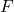belongs to a family of CDFs characterized by a parameter (vector)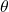. As the form ofis assumed, the target of estimation is its parameters. Thus, all uncertainty aboutis comprised of uncertainty about its parameters. Parameters are estimated by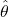, and estimates are be substituted into the assumed distribution to conduct inference for the quantities of interest. If the assumed distributionis incorrect, inference may also be inaccurate, or trends in the data may be missed.

To demonstrate the parametric approach, consider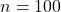independent and identically distributed random variables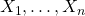generated from an exponential distribution with rate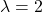. Investigators wish to estimate the 75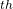percentile and erroneously assume that their data is normally distributed. Thus,is assumed to be the Normal CDF but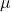and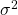are unknown. The parametersand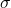are estimated in their typical way by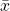and, respectively. Since the normal distribution belongs to the location-scale family, an estimate of the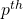percentile is provided by,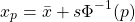where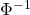is the standard normal quantile function, also known as the probit.

set.seed(12345)
library(tidyverse, quietly = T)

# Generate data from Exp(2)
x <- rexp(n = 100, rate = 2)

# True value of 75th percentile with rate = 2
true <- qexp(p = 0.75, rate = 2)
true

##  0.6931472

# Estimate mu and sigma
xbar <- mean(x)
s    <- sd(x)

# Estimate 75th percentile assuming mu = xbar and sigma = s
param_est <- xbar + s * qnorm(p = 0.75)
param_est

##  0.8792925


The true value of the 75percentile of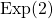is 0.69 while the parametric estimate is 0.88.

Nonparametric statistics make fewer distributions about the unknown distribution, requiring only mild assumptions such as continuity or the existence of specific moments. Instead of estimating parameters of,itself is the target of estimation.is commonly estimated by the empirical cumulative distribution function (ECDF)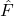,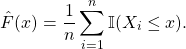Any statistic that can be expressed as a function of the CDF, known as a statistical functional and denoted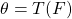, can be estimated by substitutingfor. That is, plug-in estimators can be obtained as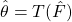.

Returning to the previous example, if nonparametric estimation is used,is estimated by the ECDF. Then, the 75percentile is estimated as the observation corresponding to the 75percentile of.

# Estimate CDF
Fhat <- ecdf(x)

# Find 75th percentile
nonparam_est <- quantile(Fhat, p = 0.75)
nonparam_est

##       75%
## 0.6174771


The nonparametric estimate of 0.62 is much closer to the true value 0.69 than the parametric estimate of 0.88.

A plot comparing the three distribution functions (true, empirical/nonparametric, and normal/parametric) is presented below to provide insight into why the nonparametric approach performed better in this scenario. Note that the exponential and normal distribution functions are continuous while the ECDF is discrete, assigning mass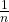to each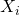.

The magnitude of the discrepancy between the parametric estimate and true value of the 75percentile can be attributed to the incorrect assumption that the data is normally distributed when it is, in fact, exponentially distributed.

The exponential distribution cannot yield negative realizations, so its CDF (blue) promptly increases starting at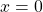, resembling logarithmic growth. On the other hand, the normal distribution can yield negative realizations, with its CDF (green) featuring non-zero probabilities for values below 0.

Comparing the two distribution functions, it is apparent that the “S”-shaped normal CDF overestimates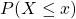when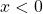or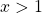while underestimating the probability of any values falling in the middle,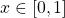. The true 75percentile 0.69 happens to fall within this range.

The nonparametric estimate is based solely on theobservations. The ECDF, therefore, 1) does not assign probability to the impossible negative values and 2) better approximates the shape of the true distribution. As a result, the nonparametric approach yields a better estimate of the 75percentile in this scenario.

# Generate sequence between -1 and 3 with step size of 0.001 for plotting.
xx <- seq(-1, 3, 0.001)

# Get values of CDFs for each xx
cdf_vals <- data.frame(Type = c(rep('True', length(xx)),
rep('Normal (parameteric)', length(xx)),
rep('Empirical (nonparametric)', length(xx))),
Support = rep(xx, 3),
CDF     = c(pexp(xx, rate = 2),
pnorm(xx, mean = xbar, sd = s),
Fhat(xx)))

# Plot comparison of CDFs
cdf_vals %>%
ggplot(aes(x = Support, y = CDF, col = Type)) +
geom_step(lwd = 1.25, alpha = 0.75) +
geom_abline(intercept = 0.75, slope = 0, lty = 2) +
labs(x = 'x', y = 'Pr(X <= x)', col = 'CDF') +
theme_bw()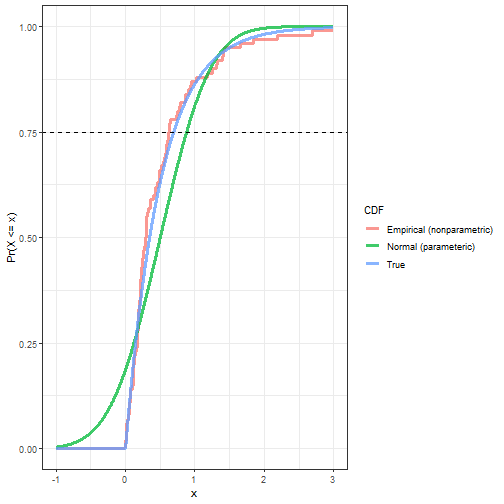What would the result have been if the investigators correctly assumed an exponential distribution?

If the rate parameter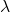is estimated using its MLE as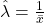, thepercentile can be estimated as,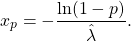# Estimate mu and sigma
lambdahat <- 1 / mean(x)
lambdahat

##  2.000546

# Estimate 75th percentile assuming mu = xbar and sigma = s
param_est2 <- -log(1 - 0.75) / lambdahat
param_est2

##  0.6929579


The parametric estimate is now much closer to the true value of 0.69 than the nonparametric estimate! In fact, the parametric estimate is basically identical to the true value.

Parametric and nonparametric methods both have their pros and cons. If the wrong distribution is assumed, parametric methods can provide flawed or misleading estimates and result in missed trends. If the correct distribution is assumed, parametric methods can leverage the knowledge of the distribution to provide precise and accurate estimates. If the investigator is unsure which assumptions to make, nonparametric methods offer a safety net by relying on observed data only and often provide reasonable estimates. However, (small) noisy samples can negatively impact the estimation of the ECDF and thus yield poor estimates of the quantities of interest.

If you have a strong hunch about the actual distribution of the data, parametric estimation is likely the way to go. But, if not, it never hurts to explore nonparametric estimation.### Emma Smith

Emma Smith is a young statistician who's on a mission to convince the masses statistics is as awesome as she *knows* it is! When she's not working on expanding her knowledge of machine learning and mathematical statistics, she's busy petting cats and unsuccessfully convincing her boyfriend to let her adopt them, hiking, concocting indie and folk rock playlists, and kicking butt in roller derby.

## 3 thoughts on “Parametric vs. Nonparametric Approach to Estimations”

1.Jackie matthews says:

on line 4, paragraph one, a word has been omitted. It should read: estimates are to be substituted…
Just saying
Jackie

1.Emma Smith says:

Haha, thank you Jackie! I will be sure to send all my drafts to you for proofreading in the future. 😛

Emma